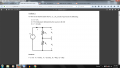# SERIES CIRCUIT, 2 RESISTORS. FIND R1, R2, V1,V2. GIVEN: POWER, V. FOUND: I.

#### HPage5

Joined Jan 17, 2016
15
Hello there, first post here. I am a Mechanical Engineering student and am taking a mandatory circuits class. This is a homework problem, but I not not fishing for the answer, I just need some help to get to the answer.

I have a series circuit with voltage source and 2 resistors. very simple circuit.

Given:
Vsource=24V
Power=48mW

Found:
Itot=P/V=2mA
Req=V/I=R1+R2=12Kohms

Still need to find individual values for R1, R2, V1, and V2.

Relevant equations:
P=IV=I^2R=V^2/R
V1=I*R1
V2=I*R2
Vs=V1+V2
V=IR
I=V/R
R=V/I
R1=V1/I
R2=V2/I
Voltage Divides.
Current is constant.

The problem before this one was a parallel circuit the same way. I found all those values after an hour of crunching. I'm trying to apply the same thinking to this one, but I feel I may be overthinking it.

I have been on this for hours, running in circles.

I have tried to manipulate the Power and Ohms Law equations every way possible to solve a system. But, it seems what I am trying to solve for always cancels out, or I end with up 1. But, I believe this would be a system with 4 unknowns, 4 equations?
Does that seem correct? Any help would be absolutely appreciated. I do not like circuits, but am trying to understand and apply the concepts.

Thanks!

#### WBahn

Joined Mar 31, 2012
29,528
With the information given, is there any way to distinguish a circuit in which R1 = 10 kΩ and R2 = 2 kΩ from a circuit in which R1 = 5 kΩ and R2 = 7 kΩ?

#### K1JOS

Joined Aug 17, 2014
12
If a DC circuit, I don't think you can deduct more than the Req related values you have done already without some additional information about individual R1 or R2 properties. There are an infinite number of R1 and R2 combinations that will give the same Req. I may be wrong and if so would love to learn more as wellJerry

#### HPage5

Joined Jan 17, 2016
15
My instructor provided the answers, being, R1=4Kohms and R2=8Kohms. V1=16V and V2=8V. or vice versa.

Does a system with 4 eqs and 4 unkns sound like the right route to take?

#### K1JOS

Joined Aug 17, 2014
12
Jerry[/QUOTE]
My instructor provided the answers, being, R1=4Kohms and R2=8Kohms. V1=16V and V2=8V. or vice versa.

That's just one of many answers.. could also be R1=6k and R2=6k and V1=V2 = 12v

#### WBahn

Joined Mar 31, 2012
29,528
My instructor provided the answers, being, R1=4Kohms and R2=8Kohms. V1=16V and V2=8V. or vice versa.

Does a system with 4 eqs and 4 unkns sound like the right route to take?
Based on what?

In order to find specific values for R1 and R2, you have to have some way of distinguishing R1 from R2. The information you have provided doesn't do that.

You mentioned a prior problem with resistors in parallel. Is it possible that the two problems are linked (i.e., both problems are using the same R1 and R2)?

#### HPage5

Joined Jan 17, 2016
15
no they are not linked. two different problems.

based on my professor wrote the problems, so that's the answers he gave us.

I was trying----

12Kohms-R2=R1

then trying to plug that R1 into---

P=I^2*Req--->P=I^2*(R1+R2)---->P/(I^2*R2)=I^2*R1---->P/(I^2*R2)=I^2(12Kohms-R2)----->P(I^2*12Kohms)/I^2R1...etc...
but, i keep working the algebra down, and R1 ends up canceling, or becoming 1 or -1.
and the same thing happens when trying to solve for other things.

#### Jony130

Joined Feb 17, 2009
5,475
As WBahn point out it is impossible to find the solution. So please post the question here.

#### HPage5

Joined Jan 17, 2016
15
Thanks for the thoughts everyone.

I forgot about a screen shot, ill try to attach one. Still new to forums. Maybe I forgot to mention something, but I think i covered it all.

I emailed my professor about it. I think, like you guys, there is information missing. He is a fan of writing convoluted questions, so maybe that's the case. Might be some mix of systems, and mesh/nodal methods to solve. But I have no idea.#### Jony130

Joined Feb 17, 2009
5,475
Hey, why didn't you mention about V2 = 2*V1 ?? And now you have that extra information, and now you can easily solve for R1 and R2.

#### HPage5

Joined Jan 17, 2016
15
I forgot about that one. I posted from memory before. My apologies. so then, try to solve V2 first and go from there?

#### HPage5

Joined Jan 17, 2016
15
I got it! all values! I cant belive i was over looking that key V2=2V1. ugh. Thank you all so much for talking me through this problem. Your help has been greatly valued.

V1=8V
V2=16V
R1=4Kohms
R2=8Kohms

thanks again you guys, you saved my sunday!